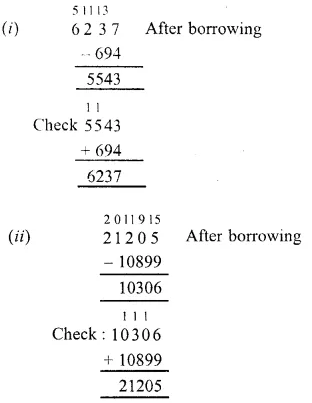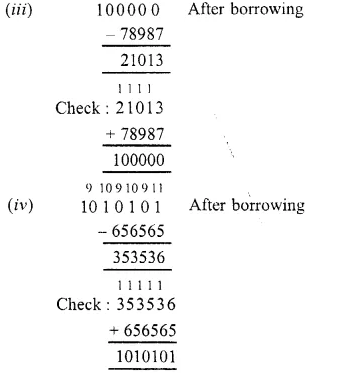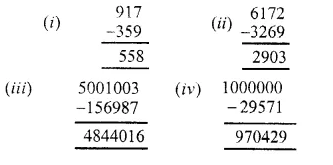## RS Aggarwal Class 6 Solutions Chapter 3 Whole Numbers Ex 3C

These Solutions are part of RS Aggarwal Solutions Class 6. Here we have given RS Aggarwal Solutions Class 6 Chapter 3 Whole Numbers Ex 3C.

Other Exercises

Question 1.
Solution:Question 2.
Solution:Question 3.
Solution:
(i) 463 – 9
= 464 – 1 – 9
= 464 – 10
= 454
(ii) 5632 – 99
= 5632 – 100 + 1
= 5633 – 100
= 5533
(iii) 8640 – 999
= 8640 – 1000 + 1
= 8641 – 1000
= 7641
(iv) 13006 – 9999
= 13006 – 10000 + 1
= 13007 – 10000
= 12007

Question 4.
Solution:
Smallest number of 7-digits = 1000000
Largest number of 4-digits = 9999
Required difference = (1000000 – 9999)
= 990001

Question 5.
Solution:
Deposit in the beginning = Rs. 136000
Next day withdrew = Rs. 73129
Amount left in the bank account = Rs. 136000 – 73129
= Rs. 62871

Question 6.
Solution:
Amount withdrawn from the bank = Rs. 1,00,000
Cost of TV set = Rs. 38750
Cost of refrigerator = Rs. 23890
Cost of jewellery = Rs. 35560
Total amount spent on her purchase = Rs. (38750 + 23890 + 35560)
= Rs. 98200
Amount left with her – Rs. 1,00,000 – Rs. 98200
= Rs. 1800

Question 7.
Solution:
Population of a town = 110500
Increase in 1 year = 3608
Persons left or died = 8973
The population at the end of year = 110500 + 3608 – 8973
= 114108 – 8973
= 105135

Question 8.
Solution:
(i) We have n + 4 = 9
n = 9 – 4 = 5
n = 5
(ii) n + 35 = 101
Subtracting 35 from both sides
n + 35 – 35 = 101 – 35
=> n = 66
n = 66
(iii) n – 18 = 39